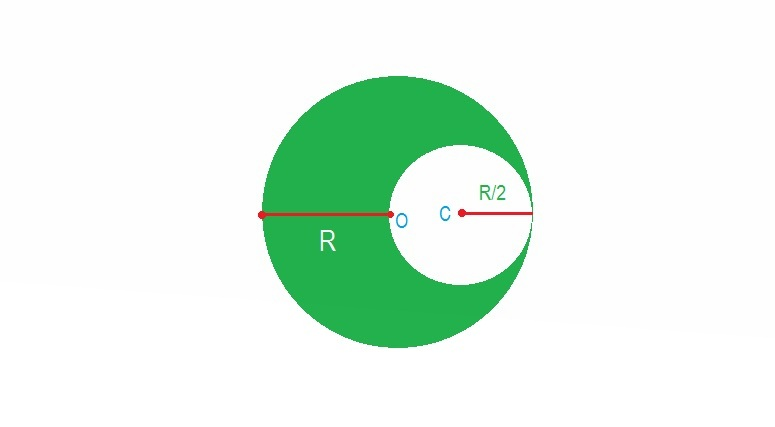G- Field of a sphereA solid sphere of uniform density with mass $M$ and radius $R$ has its center at $(0,0,0)$ which is the origin of the coordinate system. A spherical cavity of radius $\frac { R }{ 2 }$ having center at $C$ is made in the sphere by removing part of the material as shown in the figure above. Then the gravitational field intensity at $C$ is

×